Arc and it’s types:

• An arc is any part of the circumference.
• A chord divides the circumference of a circle in two parts or two arcs.
• The arc which is less than the semi-circle is called minor arc while the one that is larger then than the semi-circle is called major arc.
• A segment is the part of the circle bounded by an arc and a chord.

Theorem 9: The angle which an arc of a circle subtends at the center is double that which it subtends on any point of the part of the circumference.Given:$O$ is the center of the given circle.$\widehat{APB}$ subtends$\angle AOB$ at the center and$\angle ACB$ at a point$C$ on the circumference.

To Prove:$\angle AOB = 2 \angle ACB$

Proof: In$\triangle AOC$,$OA = OC$ (Radius of the same circle)

Therefore$\angle OAC = \angle OCA$ (angle opposite to the equal sides of a triangle are equal)$\angle AOD = \angle OAC + \angle OCA$ (exterior angle of a$\triangle =$ sum of the opposite interior angles)$= \angle OCA + \angle OCA$$= 2 \angle OCA$

Similarly in$\triangle BOC, \angle BOD = 2 \angle OCB$

Hence$\angle AOB = \angle AOD + \angle BOD$$= 2 \angle OCA + 2 \angle OCB$$= 2 ( \angle OCA + \angle OCB)$$= 2 \angle ACB$.

Hence Proved.

Similarly, if you look at the following figures you will see that: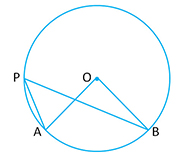i)$\angle AOB = 2 \angle APB$

ii)$Reflex \ \angle AOB = 2 \angle APB$

Theorem 10: Angles in the same segment of a circle are equal.

Given: A center with center$O$.$\angle ACB \ and \ \angle ADB$ are in the same segment of the circle.To Prove:$\angle ACB = \angle ADB$

Proof:  From Theorem 9, we know that$\angle AOB = 2 \angle ACB$ (angle at the center is twice the angle at the remaining circumference)

Similarly$\angle AOB = 2 \angle ADB$ (angle at the center is twice the angle at the remaining circumference)

This implies that$\angle ACB = \angle ADB$.

Hence Proved.

Similarly, in the adjoining figure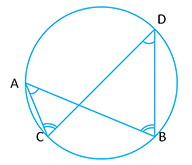i)$\angle DAB = \angle DCB$ (Angles in the same segment)

ii)$\angle ADC = \angle ABC$  (Angles in the same segment)

Theorem 11: The angle in a semi circle is a right angle.

Given: A circle with center$O$.$AB$ is the diameter and$\angle ACB$ is the angle of semi- circle.To Prove:$\angle ACB = 90^o$

Proof:$\angle AOB = 2 \angle ACB$ (angle at the center is twice the angle at the remaining circumference)

Given$\angle AOB = 180^o$ (straight line)$\Rightarrow 180^o=2 \angle ACB$$\Rightarrow \angle ACB = 90^o$.

Hence Proved.

Cyclic Properties

Theorem 12: The opposite angles of a cyclic quadrilateral inscribed in a circle are supplementary.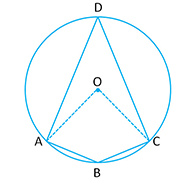Given: A circle with center$O$. A quadrilateral$ABCD$ inscribed in a circle.

To Prove:$\angle ABC + \angle ADC = 180^o \ and \ \angle BAD + \angle BCD = 180^o$

Proof:$\angle AOC = 2 \angle ADC$ (angle at the center is twice the angle at the remaining circumference)$\displaystyle \Rightarrow \angle ADC = \frac{1}{2} \angle AOC$$\displaystyle \text{ Similarly } \angle ABC = \frac{1}{2} \text{ reflex } \angle AOC$$\displaystyle \angle ABC + \angle ADC = \frac{1}{2} \angle AOC + \frac{1}{2} \text{ reflex } \angle AOC$$\displaystyle = \frac{1}{2} (\angle AOC + \text{ reflex } \angle AOC) = \frac{1}{2} \times 360^o = 180^o$$\displaystyle (\text{ Reflex } \angle AOC + \angle AOC = 360^o)$

Similarly we can prove that$\displaystyle \angle BAD + \angle BCD = 180^o$.

Hence Proved.

Note:• If the opposite angles of a quadrilateral are supplementary, then the quadrilateral is cyclic
• The angle in the major segment is acute and the angle in the minor segment is obtuse. i.e.$\angle ABC < 90^o$ while$\angle ADC > 90^o$

Theorem 13: The exterior angle of a cyclic quadrilateral is equal to the interior opposite angle.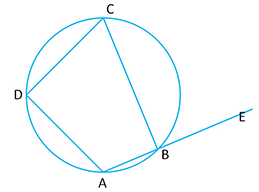Given: Circle with center$O. ABCD$ is the cyclic quadrilateral.

To Prove:$\angle CBE = \angle ADC$

Proof:$\angle ABC + \angle CBE = 180^o$ (the two angles are supplementary)$\angle ABC + \angle ADC = 180^o$(Opposite angles of a a cyclic quadrilateral are supplementary…Theorem 12)

Therefore$\angle ABC + \angle CBE = \angle ABC + \angle ADC$$\Rightarrow \angle CBE = \angle ADC$

Hence Proved.

Note: Take a look at the adjoining figure. You will see that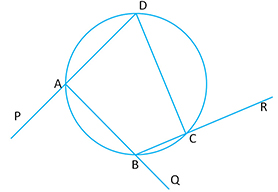i)$\angle PAB = \angle BCD$

ii)$\angle QBC = \angle ADC$

iii)$\angle RCD = \angle BAD$